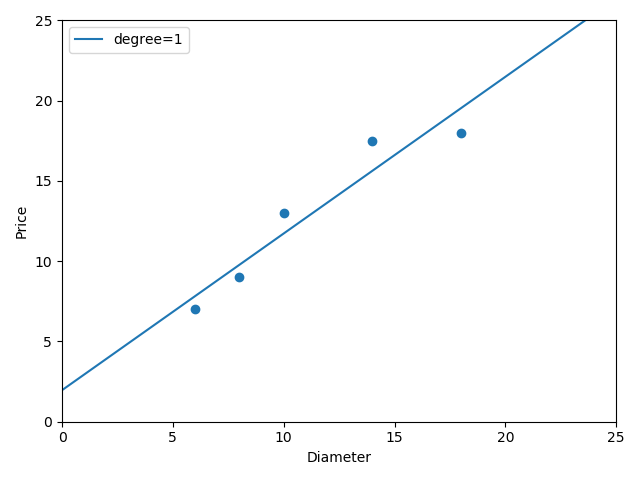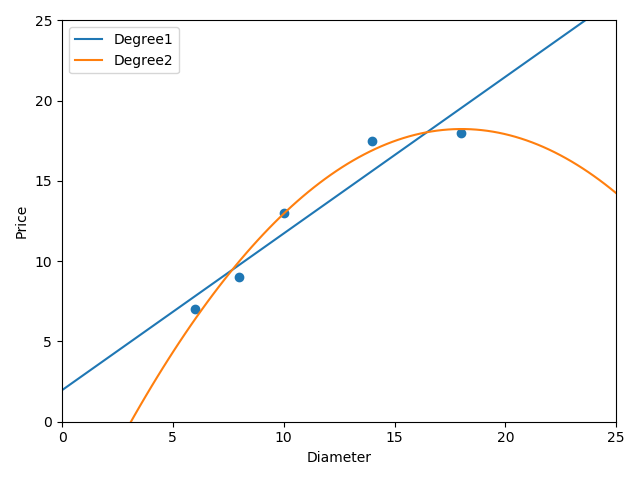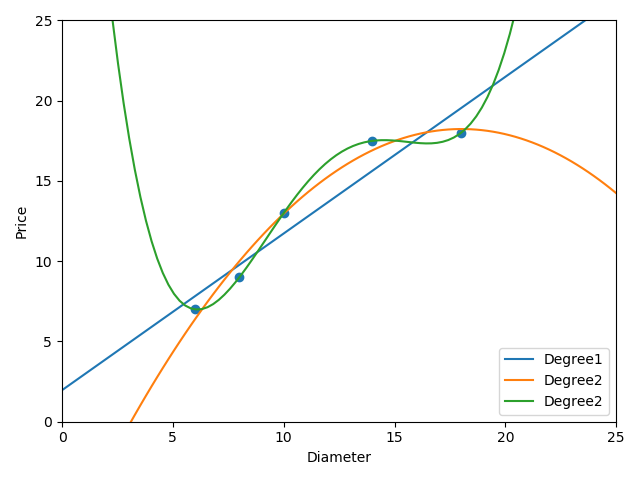• 如题，我想在线性回归的时候，考虑x，y的误差dx，dy，应该怎么做，最小二乘法好像只能考虑一个轴上的误差，但是我想同时考虑x和y的误差再拟合
• 然后洗澡的时候就在想一个问题，线性回归会了，写线性拟合是完全没问题的，但是np库的多项式拟合到底是怎么做出来的呢？突然灵光一闪多项式拟合？多变量的线性回归？好像发现了什么？重新理清一下思路。什么是多项式...
上篇博客写完之后，终于发现自己线性回归入门！然后洗澡的时候就在想一个问题，线性回归会了，写线性拟合是完全没问题的，但是np库的多项式拟合到底是怎么做出来的呢？突然灵光一闪多项式拟合？多变量的线性回归？好像发现了什么？重新理清一下思路。什么是多项式拟合？对的，这个问题以前没有好好思考过，以前的直观感觉是，不就是给一些x，y的点，让一个多项式去拟合，使得这个多项式的曲线看起来大致符合那些点。等等，好像忽略了什么，什么叫大致符合？对的，什么叫大致符合？答案已在心中了！就是让这些点距离这个拟合曲线的和最小！慢着，这其实就是线性回归的损失函数的定义嘛！最小化，损失函数！多项式多个参数怎么确定？多变量线性回归！每个一个次方项当成独立的一个变量！比如a*x^2+b*x+c这个标准的二次项，x^2当成x1，x当成x2，1当成x3！好了，整个思路有了，尝试一下实现吧！import numpy as np

def my_fit(x, y, power):
size = len(x)
c = np.ones((1, size))
x_arr = np.array(x)
x_a=x_arr.copy()
x_a.resize((1,size))
x_mat = np.append(x_a, c, axis=0)
y_arr = np.array(y)
y_arr.resize((1, size))
y_mat = np.mat(y_arr)
for i in range(2, power+1):
temp_x = x_arr**i
temp_x.resize((1, size))
x_mat = np.append(temp_x, x_mat, axis=0)
x_mat=np.mat(x_mat)
w = (x_mat * x_mat.T).I * x_mat * y_mat.T
w0=w.T
w0.resize(w0.size)
return w0

def f(x):
return 2*x ** 2 +x+3

x = np.linspace(-5, 5)
y = f(x) + 0.5*np.random.randn(len(x))  # 加入噪音
y_fit = np.polyfit(x, y, 2)  # 二次多项式拟合
print(y_fit)
w=my_fit(x,y,2)
print(w)激动人心的时候到了，看下输出：[1.99205043 1.00302882 3.08335708]
[1.99205043 1.00302882 3.08335708]竟然和np库的结果完全一样！惊了！虽说实现代码不算优美，但是基本思路没啥区别！懒得调参用梯度下降了，直接解出来。很好，还有一个疑问，scipy的曲线拟合怎么做的呢？一样嘛！当成多变量线性回归就好！code：import numpy as np
from scipy.optimize import curve_fit

def f_fit(x, a, b, c):
return a*np.sin(x)+b*x+c

def f_test(x):
return 2*np.sin(x)+3*x+1

def my_curve_fit1(fit_fun, x, y, p_num):  #p_num参数的个数
size = len(x)
if p_num <= 0 or p_num > size:
print('no parameter to fit')
return
test_list = *p_num
test_list = 1
x_arr = np.array(fit_fun(x, *test_list))
x_arr.resize((1, size))
for i in range(1, p_num):
test_list[i-1] = 0
test_list[i] = 1
temp_x = np.array(fit_fun(x, *test_list))
temp_x.resize((1, size))
x_arr = np.append(x_arr, temp_x, axis=0)
x_mat = np.mat(x_arr)
y_arr = np.array(y)
y_arr.resize((1, size))
y_mat = np.mat(y_arr)
w = (x_mat * x_mat.T).I * x_mat * y_mat.T
w0 = w.T
w0.resize(w0.size)
return w0

#为了强行接近库函数的接口,无需参数的个数，但实现有点捞
#很容易知道参数个数多于样本x的个数无法拟合
def my_curve_fit2(fit_fun, x, y):
test_list = 
size = len(x)
p_num = 1  #参数个数默认为1
for i in range(0, size):
try:
fit_fun(x, *test_list)
break
except:
p_num += 1
test_list.append(0)
if p_num > size:
print('can not fit')
return
test_list = 1
x_arr = np.array(fit_fun(x, *test_list))
x_arr.resize((1, size))
for i in range(1, p_num):
test_list[i - 1] = 0
test_list[i] = 1
temp_x = np.array(fit_fun(x, *test_list))
temp_x.resize((1, size))
x_arr = np.append(x_arr, temp_x, axis=0)
x_mat = np.mat(x_arr)
y_arr = np.array(y)
y_arr.resize((1, size))
y_mat = np.mat(y_arr)
w = (x_mat * x_mat.T).I * x_mat * y_mat.T
w0 = w.T
w0.resize(w0.size)
return w0

x = np.linspace(-2*np.pi, 2*np.pi)
y = f_test(x)+0.3*np.random.randn(len(x))   #加入噪音
p_fit, prov = curve_fit(f_fit, x, y)  #曲线拟合
my_fit1 = my_curve_fit1(f_fit, x, y, 3)
my_fit2 = my_curve_fit2(f_fit, x, y)
print('sicpy库的曲线拟合')
print('a,b,c', p_fit)
print('手写曲线拟合1')
print('a,b,c', my_fit1)
print('手写曲线拟合2')
print('a,b,c', my_fit2)输出：sicpy库的曲线拟合
a,b,c [1.99131057 3.00473361 1.05173153]
手写曲线拟合1
a,b,c [1.99131057 3.00473361 1.05173153]
手写曲线拟合2
a,b,c [1.99131057 3.00473361 1.05173153]结果竟然也就是一样！厉害了，竟然一样。好了，实现的小窍门用到了列表参数传递，想单独提出来的那个项，就令该项的参数为1，其他参数为0，然后就把每个项都单独提出来。一用多变量线性回归，搞掂！然后为了强行接近scipy库的曲线拟合的接口，不知道有几个参数拟合，怎么办呢？好像没有什么好的方法，只好用try来试了，反正传的参数个数不对就会报错的，就一个一个参数往上加咯。以上。
展开全文• 分享一下 线性回归中 欠拟合 和 过拟合怎么回事~为了解决欠拟合的情 经常要提高线性的次数建立模型拟合曲线， 次数过高会导致过拟合，次数不够会欠拟合。再建立高次函数时候，要利用多项式特征生成器 生成训练...

分享一下 线性回归中 欠拟合 和 过拟合 是怎么回事~为了解决欠拟合的情 经常要提高线性的次数建立模型拟合曲线， 次数过高会导致过拟合，次数不够会欠拟合。再建立高次函数时候，要利用多项式特征生成器 生成训练数据。下面把整个流程展示一下模拟了一个预测蛋糕价格的从欠拟合到过拟合的过程git: https://github.com/linyi0604/MachineLearning在做线性回归预测时候，为了提高模型的泛化能力，经常采用多次线性函数建立模型f = k*x + b   一次函数f = a*x^2 + b*x + w  二次函数f = a*x^3 + b*x^2 + c*x + w  三次函数。。。泛化：    对未训练过的数据样本进行预测。欠拟合:    由于对训练样本的拟合程度不够，导致模型的泛化能力不足。过拟合：    训练样本拟合非常好，并且学习到了不希望学习到的特征，导致模型的泛化能力不足。在建立超过一次函数的线性回归模型之前，要对默认特征生成多项式特征再输入给模型
poly2 = PolynomialFeatures(degree=2)    # 2次多项式特征生成器
x_train_poly2 = poly2.fit_transform(x_train)
下面模拟 根据蛋糕的直径大小 预测蛋糕价格

1 from sklearn.linear_model import LinearRegression
2 import numpy as np
3 import matplotlib.pyplot as plt
4
5 '''
6 在做线性回归预测时候，
7 为了提高模型的泛化能力，经常采用多次线性函数建立模型
8
9 f = k*x + b   一次函数
10 f = a*x^2 + b*x + w  二次函数
11 f = a*x^3 + b*x^2 + c*x + w  三次函数
12 。。。
13
14 泛化：
15     对未训练过的数据样本进行预测。
16
17 欠拟合:
18     由于对训练样本的拟合程度不够，导致模型的泛化能力不足。
19
20 过拟合：
21     训练样本拟合非常好，并且学习到了不希望学习到的特征，导致模型的泛化能力不足。
22
23
24 在建立超过一次函数的线性回归模型之前，要对默认特征生成多项式特征再输入给模型
25
26 下面模拟 根据蛋糕的直径大小 预测蛋糕价格
27
28 '''
29
30 # 样本的训练数据，特征和目标值
31 x_train = [, , , , ]
32 y_train = [, , , [17.5], ]
33
34 # 一次线性回归的学习与预测
35 # 线性回归模型 学习
36 regressor = LinearRegression()
37 regressor.fit(x_train, y_train)
38 # 画出一次线性回归的拟合曲线
39 xx = np.linspace(0, 25, 100)   # 0到16均匀采集100个点做x轴
40 xx = xx.reshape(xx.shape, 1)
41 yy = regressor.predict(xx)  # 计算每个点对应的y
42 plt.scatter(x_train, y_train)   # 画出训练数据的点
43 plt1, = plt.plot(xx, yy, label="degree=1")
44 plt.axis([0, 25, 0, 25])
45 plt.xlabel("Diameter")
46 plt.ylabel("Price")
47 plt.legend(handles=[plt1])
48 plt.show()

一次线性函数拟合曲线的结果，是欠拟合的情况：下面进行建立2次线性回归模型进行预测：

1 # 2次线性回归进行预测
2 poly2 = PolynomialFeatures(degree=2)    # 2次多项式特征生成器
3 x_train_poly2 = poly2.fit_transform(x_train)
4 # 建立模型预测
5 regressor_poly2 = LinearRegression()
6 regressor_poly2.fit(x_train_poly2, y_train)
7 # 画出2次线性回归的图
8 xx_poly2 = poly2.transform(xx)
9 yy_poly2 = regressor_poly2.predict(xx_poly2)
10 plt.scatter(x_train, y_train)
11 plt1, = plt.plot(xx, yy, label="Degree1")
12 plt2, = plt.plot(xx, yy_poly2, label="Degree2")
13 plt.axis([0, 25, 0, 25])
14 plt.xlabel("Diameter")
15 plt.ylabel("Price")
16 plt.legend(handles=[plt1, plt2])
17 plt.show()
18 # 输出二次回归模型的预测样本评分
19 print("二次线性模型在训练数据上得分:", regressor_poly2.score(x_train_poly2, y_train))     # 0.9816421639597427

二次线性回归模型拟合的曲线：
拟合程度明显比1次线性拟合的要好下面进行4次线性回归模型：

1 # 进行四次线性回归模型拟合
2 poly4 = PolynomialFeatures(degree=4)    # 4次多项式特征生成器
3 x_train_poly4 = poly4.fit_transform(x_train)
4 # 建立模型预测
5 regressor_poly4 = LinearRegression()
6 regressor_poly4.fit(x_train_poly4, y_train)
7 # 画出2次线性回归的图
8 xx_poly4 = poly4.transform(xx)
9 yy_poly4 = regressor_poly4.predict(xx_poly4)
10 plt.scatter(x_train, y_train)
11 plt1, = plt.plot(xx, yy, label="Degree1")
12 plt2, = plt.plot(xx, yy_poly2, label="Degree2")
13 plt4, = plt.plot(xx, yy_poly4, label="Degree2")
14 plt.axis([0, 25, 0, 25])
15 plt.xlabel("Diameter")
16 plt.ylabel("Price")
17 plt.legend(handles=[plt1, plt2, plt4])
18 plt.show()
19 # 输出二次回归模型的预测样本评分
20 print("四次线性训练数据上得分:", regressor_poly4.score(x_train_poly4, y_train))     # 1.0

四次线性模型预测准确率为百分之百， 但是看一下拟合曲线，明显存在不合逻辑的预测曲线，
在样本点之外的情况，可能预测的非常不准确，这种情况为过拟合之前我们一直在展示在训练集合上获得的模型评分，次数越高的模型，训练拟合越好。
下面查看一组测试数据进行预测的得分情况：

1 # 准备测试数据
2 x_test = [, , , ]
3 y_test = [, , , ]
4 print("一次线性模型在测试集合上得分:", regressor.score(x_test, y_test))   # 0.809726797707665
5 x_test_poly2 = poly2.transform(x_test)
6 print("二次线性模型在测试集合上得分:", regressor_poly2.score(x_test_poly2, y_test))   # 0.8675443656345054
7 x_test_poly4 = poly4.transform(x_test)
8 print("四次线性模型在测试集合上得分:", regressor_poly4.score(x_test_poly4, y_test))   # 0.8095880795746723

会发现，二次模型在预测集合上表现最好，四次模型表现反而不好！
这就是由于对训练数据学习的太过分，学习到了不重要的东西，反而导致预测不准确。


展开全文• 首先分析一下我们需要什么： 我们需要解决什么数学...关键是具体该怎么做？这上面三个问题对应了以下三个步骤： 按照一个或一些选出的模型类型对数据进行拟合 从一些已经拟合的类型中选取最适合的模型。 根据模型...
首先分析一下我们需要什么：
我们需要解决什么数学问题？这个问题该用什么模型？
找到一个模型使用的区别准则。这是根据1来讲的，这句话的意思，是用于体现不同模型与实际未知模型有多少偏差的准则。
同时做出预测时也要有一个判定的准则（标准）。

关键是具体该怎么做？这上面三个问题对应了以下三个步骤：
按照一个或一些选出的模型类型对数据进行拟合
从一些已经拟合的类型中选取最适合的模型。
根据模型和另外搜集到的一些数据做出预测

几个问题：线性拟合，损失函数，最小二乘与最大似然估计
所以问一个问题：什么是线性拟合？

依我看来线性拟合就是：用搜集到的几组数据分别作为自变量与因变量与你认为正确的模型进行拟合，找到一个损失函数并算出模型函数式子的过程。

所以有有人会问什么是损失函数与模型函数？

损失函数说的直白点就是用于表示计算值与实际值可能的误差大小，一般是所有误差值绝对值之和或者是平方之和，分别称为LAD回归和OLS回归。一般情况下使用的是OLS，它的普适性更高。

模型函数则是最后的表达式他是关于自变量与因变量的表达式，理论上代入x就可以预测出y。

那怎么计算误差函数呢？有两个常用方法：最小二乘和最大似然估计。这两个都是纯《概率论与数理统计》知识。

具体概念推荐同济大学的MOOC课程和数学建模第三版：

最大似然估计：

https://www.icourse163.org/learn/ZJU-232005?tid=1003251007#/learn/content?type=detail&id=1004586126&cid=1005777333

最小二乘法：

https://blog.csdn.net/qq_41938259/article/details/94557712

代码在这：

# -*- coding: UTF-8 -*-

"""

此脚本用于比较LAD线性回归和OLS线性回归

"""

import statsmodels.api as sm
from sklearn import linear_model
from statsmodels.regression.quantile_regression import QuantReg
import matplotlib.pyplot as plt
import numpy as np
import pandas as pd

def generate_data():

"""

随机生成数据

"""

np.random.seed(4889)

# Python2和Python3的range并不兼容，所以使用list(range(10, 29))
x = np.array( + list(range(10, 29)))
error = np.round(np.random.randn(20), 2)
y = x + error

# 增加异常点
x = np.append(x, 29)
y = np.append(y, 29 * 10)
return pd.DataFrame({"x": x, "y": y})

def train_OLS(x, y):

"""

训练OLS线性回归模型，并返回模型预测值

"""

model = linear_model.LinearRegression()
model.fit(x, y)
re = model.predict(x)
return re

def train_LAD(x, y):

"""

训练LAD线性回归模型，并返回模型预测值

"""

X = sm.add_constant(x)
model = QuantReg(y, X)
model = model.fit(q=0.5)
re = model.predict(X)
return re

def visualize_model(x, y, ols, lad):

"""

模型结果可视化

"""

# 创建一个图形框
fig = plt.figure(figsize=(6, 6), dpi=80)

# 在图形框里只画一幅图
ax = fig.add_subplot(111)

# 设置坐标轴
ax.set_xlabel("$x$")
ax.set_xticks(range(10, 31, 5))
ax.set_ylabel("$y$")

# 画点图，点的颜色为蓝色，半透明
ax.scatter(x, y, color="b", alpha=0.4)

# 将模型结果可视化出来
# 用红色虚线表示OLS线性回归模型的结果
ax.plot(x, ols, 'r--', label="OLS")

# 用黑色实线表示LAD线性回归模型的结果
ax.plot(x, lad, 'k', label="LAD")
plt.legend(shadow=True)

# 展示上面所画的图片。图片将阻断程序的运行，直至所有的图片被关闭
# 在Python shell里面，可以设置参数"block=False"，使阻断失效
plt.show()

def OLS_vs_LAD(data):

"""

比较OLS模型和LAD模型的差异

"""

features = ["x"]
label = ["y"]
ols = train_OLS(data[features], data[label])
lad = train_LAD(data[features], data[label])
visualize_model(data[features], data[label], ols, lad)

if __name__ == "__main__":
data = generate_data()
OLS_vs_LAD(data)

END


展开全文• 常见机器学习算法名单 1.线性回归 线性回归通常用于根据连续变量估计实际数值...假设在不问对方体重的情况下，让一个五年级的孩子按体重从轻到重的顺序对班上的同学排序，你觉得这个孩子会怎么做？他（她）很可能
常见机器学习算法名单
1.线性回归
线性回归通常用于根据连续变量估计实际数值（房价、呼叫次数、总销售额等）。我们通过拟合最佳直线来建立自变量和因变量的关系。这条最佳直线叫做回归线，并且用 Y= a *X + b 这条线性等式来表示。
理解线性回归的最好办法是回顾一下童年。假设在不问对方体重的情况下，让一个五年级的孩子按体重从轻到重的顺序对班上的同学排序，你觉得这个孩子会怎么做？他（她）很可能会目测人们的身高和体型，综合这些可见的参数来排列他们。这是现实生活中使用线性回归的例子。实际上，这个孩子发现了身高和体型与体重有一定的关系，这个关系看起来很像上面的等式。
在这个等式中：Y：因变量 a：斜率 x：自变量  b ：截距
系数 a 和 b 可以通过最小二乘法获得。线性回归的两种主要类型是一元线性回归和多元线性回归。一元线性回归的特点是只有一个自变量。多元线性回归的特点正如其名，存在多个自变量。找最佳拟合直线的时候，你可以拟合到多项或者曲线回归。这些就被叫做多项或曲线回归
Python 代码
from sklearn import linear_model
x_train=input_variables_values_training_datasets
y_train=target_variables_values_training_datasets
x_test=input_variables_values_test_datasets
linear = linear_model.LinearRegression()
linear.fit(x_train, y_train)
linear.score(x_train, y_train)
print('Coefficient: n', linear.coef_)
print('Intercept: n', linear.intercept_)
predicted= linear.predict(x_test)
2.逻辑回归
别被它的名字迷惑了！这是一个分类算法而不是一个回归算法。该算法可根据已知的一系列因变量估计离散数值（比方说二进制数值 0 或 1 ，是或否，真或假）。简单来说，它通过将数据拟合进一个逻辑函数来预估一个事件出现的概率。因此，它也被叫做逻辑回归。因为它预估的是概率，所以它的输出值大小在 0 和 1 之间（正如所预计的一样）。
让我们再次通过一个简单的例子来理解这个算法。
假设你的朋友让你解开一个谜题。这只会有两个结果：你解开了或是你没有解开。想象你要解答很多道题来找出你所擅长的主题。这个研究的结果就会像是这样：假设题目是一道十年级的三角函数题，你有 70%的可能会解开这道题。然而，若题目是个五年级的历史题，你只有30%的可能性回答正确。这就是逻辑回归能提供给你的信息。
从数学上看，在结果中，几率的对数使用的是预测变量的线性组合模型。
odds= p/ (1-p) = probability of event occurrence / probability of not event occurrence
ln(odds) = ln(p/(1-p))
logit(p) = ln(p/(1-p)) = b0+b1X1+b2X2+b3X3....+bkXk
p 是我们感兴趣的特征出现的概率。
Python代码
from sklearn.linear_model import LogisticRegression
model = LogisticRegression()
model.fit(X, y)
model.score(X, y)
print('Coefficient: n', model.coef_)
print('Intercept: n', model.intercept_)
predicted= model.predict(x_test)
3.决策树
这个监督式学习算法通常被用于分类问题。令人惊奇的是，它同时适用于分类变量和连续因变量。在这个算法中，我们将总体分成两个或更多的同类群。这是根据最重要的属性或者自变量来分成尽可能不同的组别
Python代码
from sklearn import tree
model = tree.DecisionTreeClassifier(criterion='gini')
model.fit(X, y)
model.score(X, y)
predicted= model.predict(x_test)
4.SVM
大名鼎鼎的SVM，想必大家都很熟悉了吧。这是一种分类方法。在这个算法中，我们将每个数据在N维空间中用点标出（N是你所有的特征总数），每个特征的值是一个坐标的值。
Python代码
from sklearn import svm
tor) and Y (target) for training data set and x_test(predictor) of test_dataset
model = svm.svc()
model.fit(X, y)
model.score(X, y)
predicted= model.predict(x_test)
5.朴素贝叶斯
在预示变量间相互独立的前提下，根据贝叶斯定理可以得到朴素贝叶斯这个分类方法。用更简单的话来说，一个朴素贝叶斯分类器假设一个分类的特性与该分类的其它特性不相关。朴素贝叶斯模型易于建造，且对于大型数据集非常有用。虽然简单，但是朴素贝叶斯的表现却超越了非常复杂的分类方法。
Python代码
from sklearn.naive_bayes import GaussianNB
model.fit(X, y)
predicted= model.predict(x_test)
6.K最近邻算法
该算法可用于分类问题和回归问题。然而，在业界内，K – 最近邻算法更常用于分类问题。K – 最近邻算法是一个简单的算法。它储存所有的案例，通过周围k个案例中的大多数情况划分新的案例。根据一个距离函数，新案例会被分配到它的 K 个近邻中最普遍的类别中去。这些距离函数可以是欧式距离、曼哈顿距离、明式距离或者是汉明距离。前三个距离函数用于连续函数，第四个函数（汉明函数）则被用于分类变量。如果 K=1，新案例就直接被分到离其最近的案例所属的类别中。有时候，使用 KNN 建模时，选择 K 的取值是一个挑战。
Python代码
from sklearn.neighbors import KNeighborsClassifier
KNeighborsClassifier(n_neighbors=6)
model.fit(X, y)
predicted= model.predict(x_test)
7.K均值算法
K – 均值算法是一种非监督式学习算法，它能解决聚类问题。使用 K – 均值算法来将一个数据归入一定数量的集群（假设有 k 个集群）的过程是简单的。一个集群内的数据点是均匀齐次的，并且异于别的集群。
K – 均值算法给每个集群选择k个点。这些点称作为质心。每一个数据点与距离最近的质心形成一个集群，也就是 k 个集群。根据现有的类别成员，找出每个类别的质心。现在我们有了新质心。当我们有新质心后，重复步骤 2 和步骤 3。找到距离每个数据点最近的质心，并与新的k集群联系起来。重复这个过程，直到数据都收敛了，也就是当质心不再改变。
K – 均值算法涉及到集群，每个集群有自己的质心。一个集群内的质心和各数据点之间距离的平方和形成了这个集群的平方值之和。同时，当所有集群的平方值之和加起来的时候，就组成了集群方案的平方值之和。
我们知道，当集群的数量增加时，K值会持续下降。但是，如果你将结果用图表来表示，你会看到距离的平方总和快速减少。到某个值 k 之后，减少的速度就大大下降了。在此，我们可以找到集群数量的最优值。
Python代码
from sklearn.cluster import KMeans
k_means = KMeans(n_clusters=3, random_state=0)
model.fit(X)
predicted= model.predict(x_test)
8.随机森林算法
随机森林是表示决策树总体的一个专有名词。在随机森林算法中，我们有一系列的决策树（因此又名“森林”）。为了根据一个新对象的属性将其分类，每一个决策树有一个分类，称之为这个决策树“投票”给该分类。这个森林选择获得森林里（在所有树中）获得票数最多的分类。
每棵树是像这样种植养成的：如果训练集的案例数是 N，则从 N 个案例中用重置抽样法随机抽取样本。这个样本将作为“养育”树的训练集。假如有 M 个输入变量，则定义一个数字 m<<M。m 表示，从 M 中随机选中 m 个变量，这 m 个变量中最好的切分会被用来切分该节点。在种植森林的过程中，m 的值保持不变。尽可能大地种植每一棵树，全程不剪枝。
Python代码
library(randomForest)
x <- cbind(x_train,y_train)
fit <- randomForest(Species ~ ., x,ntree=500)
summary(fit)
predicted= predict(fit,x_test)
9.降维算法
Python代码
from sklearn import decomposition
train_reduced = pca.fit_transform(train)
test_reduced = pca.transform(test)
10.Gradient Boost 和 Adaboost 算法
当我们要处理很多数据来做一个有高预测能力的预测时，我们会用到 GBM 和 AdaBoost 这两种 boosting 算法。boosting 算法是一种集成学习算法。它结合了建立在多个基础估计值基础上的预测结果，来增进单个估计值的可靠程度。这些 boosting 算法通常在数据科学比赛如 Kaggl、AV Hackathon、CrowdAnalytix 中很有效。
Python代码
from sklearn.ensemble import GradientBoostingClassifier
model= GradientBoostingClassifier(n_estimators=100, learning_rate=1.0, max_depth=1, random_state=0)
model.fit(X, y)
predicted= model.predict(x_test)

展开全文•   还记得梯度下降法是怎么做的吗？忘记的同学可以回头看下前面的博客线性回归。咱们还是拿之前博客线性回归python实现里的数据进行讲解。   假设咱们现在想用y=θ1⋅x+θ0y=θ1⋅x+θ0y=\the...机器学习
• 1、线性回归 线性回归通常用于根据连续变量估计实际数值（房价、呼叫次数、总销售额等）。...假设在不问对方体重的情况下，让一个五年级的孩子按体重从轻到重的顺序对班上的同学排序，你觉得这个孩子会怎么做...
• 实战项目 3 优化 过拟合和欠拟合 实战项目 4 古诗词自动生成 切分（分词） 词性标注 命名实体识别 句法分析 WordNet可以被看作是一个同义词词典 词干提取（stemming）与词形还原（lemmatization） ...
• 实战项目 3 优化 过拟合和欠拟合 实战项目 4 古诗词自动生成 切分（分词） 词性标注 命名实体识别 句法分析 WordNet可以被看作是一个同义词词典 词干提取（stemming）与词形还原（lemmatization） ...
• 和AdaBoost的主要区别就在于AdaBoost是在每一次迭代中修改样本权重来使得后一次的树模型更加关注被分错的样本，而GBDT则是后一次树模型直接去拟合残差。 Task11：XGBoost算法分析与案例调参实例（2天） XGBoost是一...
• 实战项目 3 优化 过拟合和欠拟合 实战项目 4 古诗词自动生成 切分（分词） 词性标注 命名实体识别 句法分析 WordNet可以被看作是一个同义词词典 词干提取（stemming）与词形还原（lemmatization） ...adaboost apriori fp-growth
• 神经网络训练如何解决过拟合和欠拟合 L1正则化和L2正则化区别，具体有何用途 L1正则化相比于 L2正则化为何具有稀疏解 2. C++开发相关 c++常见容器，vector容器capacity和size区别，如何动态增长 vector遍历有...# python线性拟合怎么做python 订阅